# Finding the Median

Finding the Median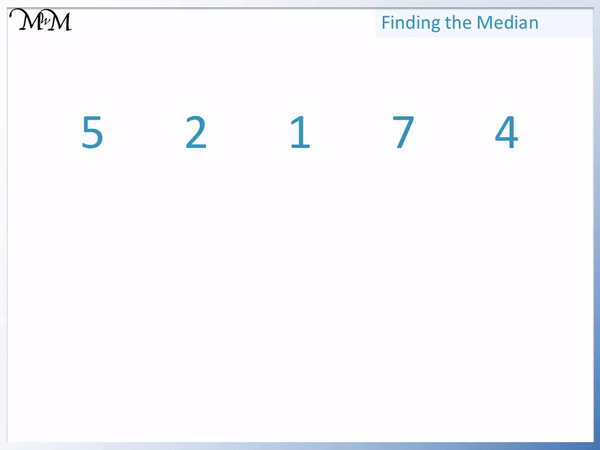• The median is a type of average.
• The median is the middle number in an ordered list of numbers.
• We first put the list of numbers in order from smallest to largest.
• The median is the middle number in this ordered list.
• To find the middle number, cross off a number on both the left and right of the list.
• Cross off numbers at each end of the list until only one number remains.

The median is middle number in a list that has been put in order from smallest to largest.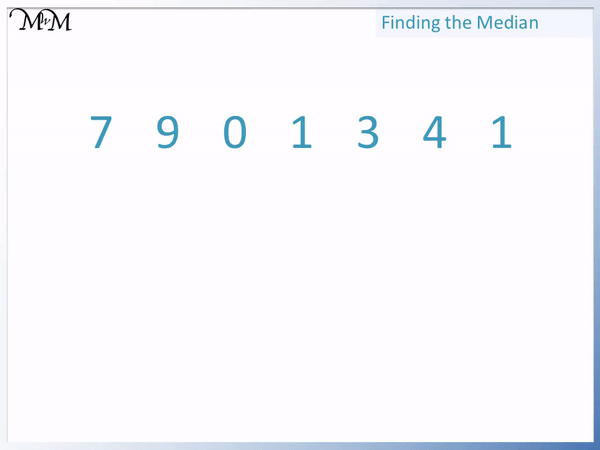• The median is the middle number in an ordered list of numbers.
• We first write the 7 numbers in order from smallest to largest.
• The list 7, 9, 0, 1, 3, 4, 1 is written in order as 0, 1, 1, 3, 4, 7, 9.
• We check that there are 7 numbers in the new ordered list so that we know that we haven’t missed any out.
• We now cross off numbers at each end of the list until one remains in the middle.
• 3 is the median of this list of numbers.Supporting Lessons# Finding the Median

## What is the Median?

The median is a type of average. The median is the middle value in an ordered list of numbers. Simply put, there are the same amount of numbers that are less than the median as there are numbers larger than the median.

For example, here is an unordered list of numbers. We first write them in order from smallest to largest.The median is the middle number in this ordered list.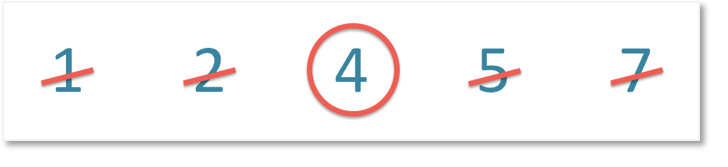The median is 4.

We can see that there are 2 numbers less than 4 and 2 numbers more than 4. The median is exactly in the middle of the data.

## How to Calculate the Median

To calculate the median use the following steps:

1. Write the numbers in order from smallest to largest.
2. Cross off a number at each end of the list.
3. Keep crossing off numbers until only one number remains.
4. If one number remains, this is the median.
5. If two numbers remain, add the numbers and divide by 2 to find the median.
6. Here is an example of calculating the median of a list of numbers.

We have the list of numbers: 5, 2, 1, 7, 4.

The first step is to write them in order from smallest to largest.

There are 5 numbers in the original list and so, there should still be 5 numbers in the reordered list.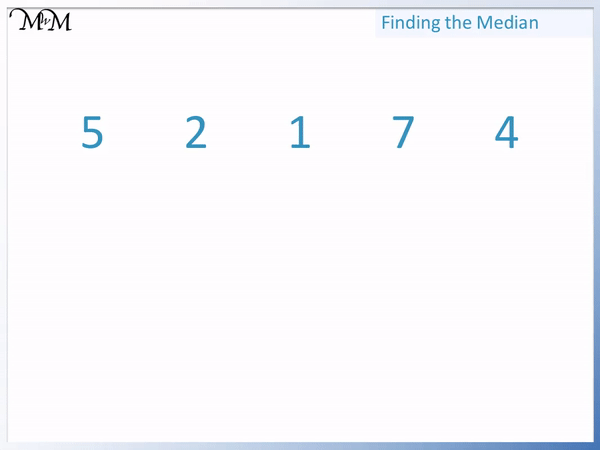Putting the list in order, we have: 1, 2, 4, 5, 7. This is still 5 numbers in total so we know that we haven’t missed any out.

When teaching the median, we should remember to count the numbers in the each list as it is a common mistake to miss out a number when reordering longer lists.

The next step is to cross off numbers at each end of the list until only one number remains.

We cross off 1 and 7 together and then 2 and 5 together.We can see that the middle number of 1, 2, 4, 5, 7 is 4.

The median of the list of numbers is 4.

Here is an animation showing the complete process of finding the median.Here is another example of finding the median of a list of numbers.

We have the list of numbers: 7, 9, 0, 1, 3, 4, 1.

The first step is to put the numbers in order making sure that there are still the same amount of numbers in the original list as there are in the reordered list.

It is also useful to identify any duplicate numbers. There are two 1’s in our list, so there should be two 1’s in the reordered list.The list in order from smallest to largest is 0, 1, 1, 3, 4, 7, 9.

There are 7 numbers in the original list and in the new reordered list, so it is likely that we haven’t missed any numbers out.

We now cross off numbers from each end of the list.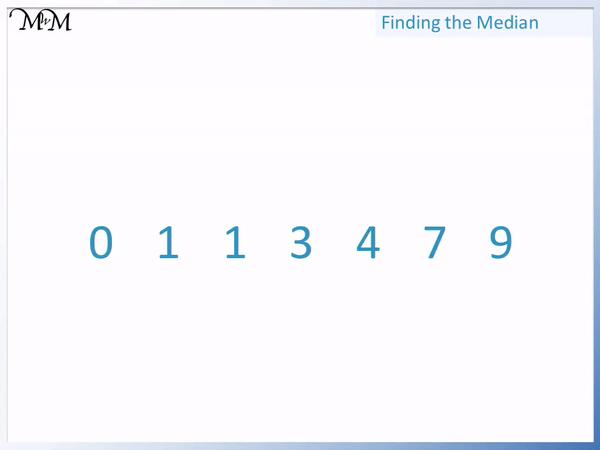We cross off numbers at each end until only one number remains.

We can see that in this list: 0, 1, 1, 3, 4, 7, 9 that 3 is the number left in the middle.

3 is the median of this list of numbers.

We can see that there are 3 numbers less than the median and 3 numbers more than the median.

Here is an animation showing how to find the median of this list of numbers.## Finding the Median with an Even Set of Numbers

If there is an even amount of numbers in the list, then two numbers remain when numbers are crossed off at each end of the list. Add these two numbers and divide the result by two to find the median.

For example here is the list: 1, 3, 4, 6, 7, 9.

There are 6 numbers, which is an even amount.

If we cross off a number at each end we have: 1, 3, 4, 6, 7, 9.

If we cross off another number at each end we have: 1, 3, 4, 6, 7, 9.

There are two numbers remaining.

If we cross off another two numbers, then all of the numbers would be crossed out.The two numbers remaining are 4 and 6.

The median is found by adding the two numbers and then dividing by 2.

4 + 6 = 10 and 10 ÷ 2 = 5.

The median is 5. 5 is directly in between 4 and 6.

To look at further examples of finding the median of an even set of numbers in more detail, look at our next lesson.Now try our lesson on Median of an Even Set of Numbers where we learn how to find the median when we have an even amount of numbers.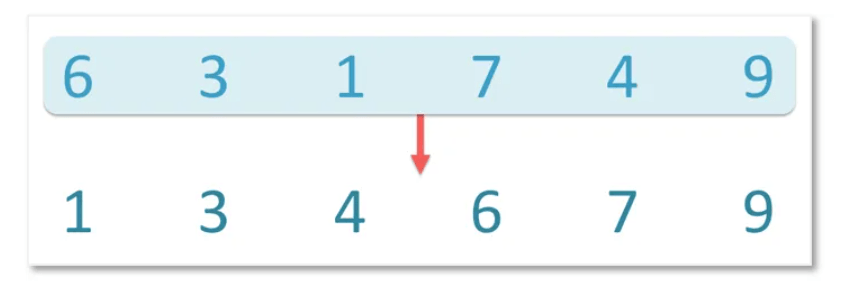error: Content is protected !!